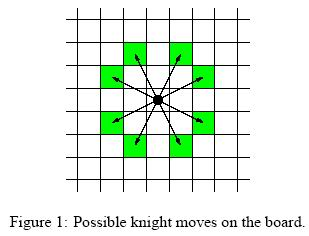# poj 1915 Knight Moves

2014/11/29 13:42

Knight Moves
 Time Limit: 1000MS Memory Limit: 30000K Total Submissions: 22220 Accepted: 10384

Description

Background
Mr Somurolov, fabulous chess-gamer indeed, asserts that no one else but him can move knights from one position to another so fast. Can you beat him?
The Problem
Your task is to write a program to calculate the minimum number of moves needed for a knight to reach one point from another, so that you have the chance to be faster than Somurolov.
For people not familiar with chess, the possible knight moves are shown in Figure 1.Input

The input begins with the number n of scenarios on a single line by itself.
Next follow n scenarios. Each scenario consists of three lines containing integer numbers. The first line specifies the length l of a side of the chess board (4 <= l <= 300). The entire board has size l * l. The second and third line contain pair of integers {0, ..., l-1}*{0, ..., l-1} specifying the starting and ending position of the knight on the board. The integers are separated by a single blank. You can assume that the positions are valid positions on the chess board of that scenario.

Output

For each scenario of the input you have to calculate the minimal amount of knight moves which are necessary to move from the starting point to the ending point. If starting point and ending point are equal,distance is zero. The distance must be written on a single line.

Sample Input

3
8
0 0
7 0
100
0 0
30 50
10
1 1
1 1

Sample Output

5
28
0

Source

TUD Programming Contest 2001, Darmstadt, Germany

bfs用sdl的list写，效率堪忧。换成queue。

#include <cstdio>
#include <cstdlib>
#include <queue>
#include <cstring>
using namespace std;
#define MAXN 303

int size = 0;
bool visit[MAXN][MAXN] = {0};

int mx = {-2, -1, 1, 2, 2, 1, -1, -2};
int my = {1, 2, 2, 1, -1, -2, -2, -1};

int step_num = 0;

struct point
{
int x;
int y;
int n;
};

queue <point> myq;
point sp;
point dp;

void init()
{
memset (visit, 0, sizeof(visit));
while(!myq.empty())
{
myq.pop();
}
return;
}

bool bfs(point p)
{
int x = p.x;
int y = p.y;
int n = p.n;

if (x < 0 || x >= size || y < 0 || y >= size)
return false;

if (visit[x][y])
return false;
visit[x][y] = true;

if (x == dp.x && y == dp.y){
step_num = n;
return true;
}

for (int i = 0; i < 8; ++i)
{
point newp;
newp.x = x + mx[i];
newp.y = y + my[i];
newp.n = n + 1;
myq.push(newp);
//printf("%d %d %d\n", newp.x, newp.y, newp.n);
}
return false;
}

int main()
{
int n = 0;

scanf("%d", &n);
for (int i = 0; i < n; ++i)
{
scanf("%d", &size);
scanf("%d%d%d%d", &sp.x, &sp.y, &dp.x, &dp.y);

init();
myq.push(sp);
while(!myq.empty())
{
if(bfs(myq.front()))
break;
myq.pop();
}
printf("%d\n", step_num);
}

}

0
0 收藏

### 作者的其它热门文章0 评论
0 收藏
0